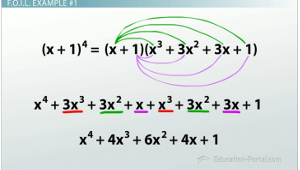# What is the Binomial Theorem?

Lesson Transcript
Instructor: Luke Winspur

Luke has taught high school algebra and geometry, college calculus, and has a master's degree in education.

While the F.O.I.L. method can be used to multiply any number of binomials together, doing more than three can quickly become a huge headache. Luckily, we've got the Binomial Theorem and Pascal's Triangle for that! Learn all about it in this lesson.

## Understanding the F.O.I.L Technique

When I tell people that I'm a high school math teacher, they often share with me what bits of their algebra class they remember. Along with the quadratic formula, the topic I hear the most about is F.O.I.L. It's an acronym that stands for First, Outer, Inner, Last and allows us to multiply two expressions like (x-3)(x+5) by multiplying the first two terms, the outer terms, the inner terms and, finally, the last terms. After we've gotten those things, we simply combine the like terms left in our expression to get our answer,x^2 + 2x - 15.

What F.O.I.L. helps us do is multiply two binomials together to get a trinomial. We begin with binomials because each expression has only two terms, and our answer is a trinomial because it has three terms. Just like a bicycle has two wheels and a tricycle has three!

An error occurred trying to load this video.

Try refreshing the page, or contact customer support.

Coming up next: Binomial Theorem Practice Problems

### You're on a roll. Keep up the good work!

Replay
Your next lesson will play in 10 seconds
• 0:06 Understanding the FOIL…
• 1:07 Example #1
• 3:08 Binomial Theorem
• 3:58 Pascal's Triangle
• 6:24 A More Complex Equation
• 8:34 Lesson Summary
Save Save

Want to watch this again later?

Log in or sign up to add this lesson to a Custom Course.

Timeline
Autoplay
Autoplay
Speed Speed## F.O.I.L. Example #1

What most people forget is that F.O.I.L. can also help us multiply expressions such as (x + 1)^3. You must first make sure not to make the common mistake of distributing the exponent to both terms on the inside, and instead re-write the expression as (x + 1)(x + 1)(x + 1). We can now begin the process of multiplying these by using F.O.I.L. to multiply the first two binomials as we normally would. We do the firsts, the outers, the inners, the lasts. We combine like terms, and we get x^2 + 2x + 1.

But, we still have another x + 1 hanging out on the end. Now it's time for what I often call the super F.O.I.L. We still need to multiply everything in the first set of parenthesis with everything in the second, but now that now requires more steps. We'll first multiply everything in the trinomial with x((x^2 * x), (2x * 1)) and (1 * x)). We'll then multiply everything in the trinomial with 1 ((x^2 * 1), (2x * 1) and (1 * 1)). Again, we have to combine like terms, and we find that (x + 1)^3 = x^3 + 3x^2 + 3x + 1.

This process can be continued for as long as you would like. For example, if we instead wanted to find (x + 1)^4, we could simply multiply our previous answer by x + 1 another time. This now becomes what you might call the super duper F.O.I.L. and requires even more steps. But it's still the same process. If we multiply everything from the first expression (the x + 1) with everything from the second expression (our answer from the last question) and then we combine all our like terms, we'll end up with (x + 1)^4 = x^4 + 4x^3 + 6x^2 + 4x+1.

## The Binomial Theorem

But as is now becoming clear, this method requires quite a bit of work. Each time we do it this way there are more and more steps for us to do, and also more opportunities for us to make a silly mistake. Because of this, just the thought of using this method for multiplying, I don't know, (x + 1)^8 gives me a headache. Luckily, we have the Binomial Theorem to save us all the money we would have had to spend on Advil.

The binomial theorem is all about patterns. Maybe you noticed that each answer we got began with an x to the same power as in our original problem. (x + 1)^3 began with an x^3. After that, the exponent on the xs went down by 1 until we got to no more xs and simply the number 1. That pattern alone tells us that (x + 1)^8 will look something like x^8 + x^7 + … down to 1. The only things we're missing are the coefficients in front of all those xs. This pattern is definitely more complicated to see, but that's exactly why French mathematician Blaise Pascal got his named attached to it when he found it ( Pascal's Triangle).

To unlock this lesson you must be a Study.com Member.
Create your account

### Register to view this lesson

Are you a student or a teacher?

### Unlock Your Education

#### See for yourself why 30 million people use Study.com

##### Become a Study.com member and start learning now.
Back
What teachers are saying about Study.com
Create an account to start this course today
Used by over 30 million students worldwide